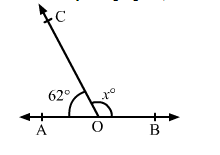# In the adjoining figure, AOB is a straight line. Find the value of x.

Question:

In the adjoining figure, AOB is a straight line. Find the value of x.Solution:

We know that the sum of angles in a linear pair is $180^{\circ}$.

Therefore,

$\angle A O C+\angle B O C=180^{\circ}$

$\Rightarrow 62^{\circ}+x^{\circ}=180^{\circ}$

$\Rightarrow x^{\circ}=\left(180^{\circ}-62^{\circ}\right)$

$\Rightarrow x=118^{\circ}$

Hence, the value of $x$ is $118^{\circ}$.